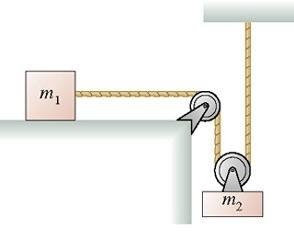# Quick forces conceptual question

## Homework StatementFind an expression for the acceleration of m1.

## The Attempt at a Solution

I did this earlier, and looking back on it I have a question:

Sum of forces for m1:
Fx= F2 on 1= m1a1

Sum of forces for m2:
Fy = F1 on 2 + T1 - m2g = m2a2

I got the expression after substituting them into each other and saying a2 = -.5a1, I'm just wondering why you can say T1= F1 on 2 , because since there is acceleration, can you consider the tension throughout the rope to be the same?

It's a double pulley problem with m1 sitting on a table, attached to a rope that goes around a pulley at the edge of the table. Then the string passes through another pulley attached to the top of a suspended m2. Then the rope goes up and is attached to a ceiling.

Doc Al
Mentor
Sum of forces for m1:
Fx= F2 on 1= m1a1
The only horizontal force on m1 is the rope tension T.

Sum of forces for m2:
Fy = F1 on 2 + T1 - m2g = m2a2
Careful. The tension force pulls up twice on m2 (via the pulley).

I got the expression after substituting them into each other and saying a2 = -.5a1, I'm just wondering why you can say T1= F1 on 2 , because since there is acceleration, can you consider the tension throughout the rope to be the same?
As long as the rope is massless and the pulley is massless and frictionless, then the tension is the same throughout the rope.